# How to find stocks on the move with a better momentum indicator - exponential regression

Has a friend ever forced you to read a book? It happened to me and I was glad he did because it showed me a better momentum indicator (exponential regression) that we immediately added to the Quant Investing stock screener.

The book he forced me to read was Stocks on the Move: Beating the Market with Hedge Fund Momentum Strategies by Andreas Clenow.

It is a great book that is easy and fun to read in spite of its intimidating title.

You can read more about the author Andreas and exponential momentum in this interview:

More insights from Andreas Clenow author of Stocks on the Move: Beating the Market with Hedge Fund Momentum Strategies

We did our own back test with the adjusted slope indicator on stocks world-wide in this article:

This easy to use adjusted slope momentum strategy performed 7 times better than the market

### A great trading strategy and better momentum

The book describes a very profitable trading strategy that uses a different way of calculating stock price momentum, to help you find stocks that are moving up (stocks on the move).

### Problem with existing momentum calculations

If you are looking for stocks that are moving up nice and smoothly there is a problem with the way momentum is normally calculated.

For example 6 months momentum is calculated as the current share price divided by the share price six months ago.

The following chart shows the problem with this calculation: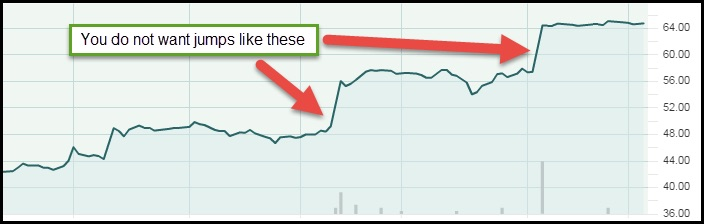You do not want jumps like this when using momentum

When looking for a stock with good momentum you want to find one that is moving up smoothly, you do not want to see large jumps in the share price, for example because of a takeover offer or better than expected results.

A stock price that makes large jumps may not move up much more because the news is already priced in.

This is not nearly as profitable as a smoothly upward moving stock price due to every day interest by investors willing to buy the stock at a slightly higher price.

## The better momentum calculation

To find stocks with a smoothly upward moving stock price you want to calculate the average daily percent increase in the stock price. To do this Andreas uses exponential regression

For example, using exponential regression, you can calculate that over a 90 day period a stock price increased by 0.05% per day.

Then, if you assume there are 260 trading days in a year, theoretically the share price may go up 13.88% (0.05% to the power 260) over a year.

Keep in mind that you do not expect to make this return but it gives you an idea of how much the stock price can increase over a year if you assume the increase over the past 90 days continues.

### All the calculations are done for you

Do not worry if the calculations look intimidating, you do not need to know any of it to use this better momentum indicator to find investment ideas.

All the calculations are done for you in the Quant Investing stock screener.

### How well does it fit - Adjusted Slope using R2

To get rid of companies with a large jump in share price (bad momentum) you want to make sure that the calculated daily price increase is as close as possible to the real daily increase in the stock price.

Luckily there is a value that allows us to do exactly that.

It is called R2 (the coefficient of determination) and it tells you how well the calculated daily stock price increase fits the real daily increase.

If Ris equal to 1 the real daily increase is exactly equal to the calculated value and if it is close to zero the calculated value is not at all close to the real daily increase.

To see how well the daily stock price increase fits the actual share price movements is easy to calculate.

Simply multiply the calculated increase (exponential regression) by the Rvalue.

If the fit is good (close to 1) the calculated value will remain the same but if it is bad (Rless than 1) the calculated share price increase will decrease.

In the screener we call the indicator (exponential regression x R2) the Adjusted Slope of the stock price.

### Adjusted Slope in the screener

The screener has two adjusted slope indicators you can use:

The Adjusted Slope 90d is equal to the annualized exponential regression slope multiplied by the coefficient of determination (R2) over the past 90 trading days

### What is means in easy to understand English

In easy to understand English, the Adjusted Slope 90d shows you how much the share price of a stock could increase over a year if you assume the increase over the past 90 trading days continues for the remainder of the year. AND this value is adjusted for how well the calculated increase matches the real increase in the stock price, as explained above.

### Where can you find it in the screener?

In the screener you can use the Adjusted Slope 90d indicator either as a primary factor or as a column in the results of your screen.How to select Adjusted Slope 90d as a primary screening factor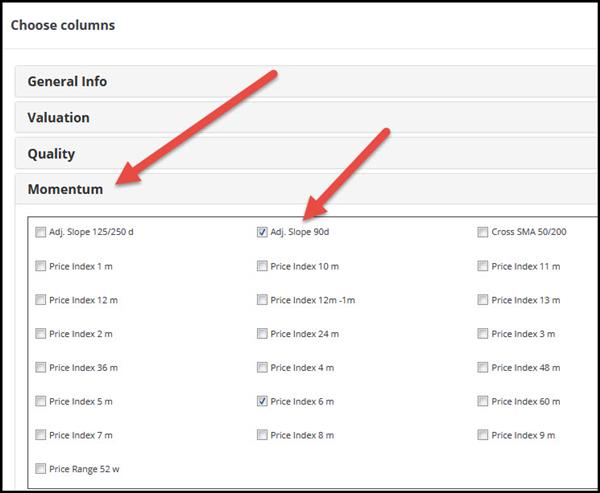How to select Adjusted Slope 90d as an output column

### What is good and bad momentum?

The higher the Adjusted Slope 90d value the better as it means the share price has increased a lot over the past 90 trading days and that the calculated increase in the stock price closely fits the real increase in the stock price.

You can select the best Adjusted Slope 90d value companies by setting the slider from 0% to 10% or by sorting the output column from high to low (to sort the column click on the column heading).How to select best Adjusted Slope 90d values

Adjusted Slope 125/250d is equal to the average annualized exponential regression slope, over the past 125 and 250 trading days, multiplied by the coefficient of determination (R2).

It is thus the average of the 125 day and 250 day adjusted slope values.

### Why an average of 125 and 250 days?

Why calculate the average you may be thinking. This is to give you a longer term (6 months and one year) average adjusted slope value that smooths out large movements in stock prices (by using the average).

You can use this indicator to find stocks that have been on a smooth upward trend over the past six months as well as the past year.

### What is means in easy to understand English

In easy to understand English, the Adjusted Slope 125/250d shows you how much the share price of a stock has increased over the past year if you average the 125d and 250d adjusted slope values. AND this value is adjusted for how well the calculated increase matches the real increase in the stock price, as explained above.

### Where can you find it?

In the screener you can use the Adjusted Slope 125/250d indicator either as a primary factor or as a column in the results of your screen.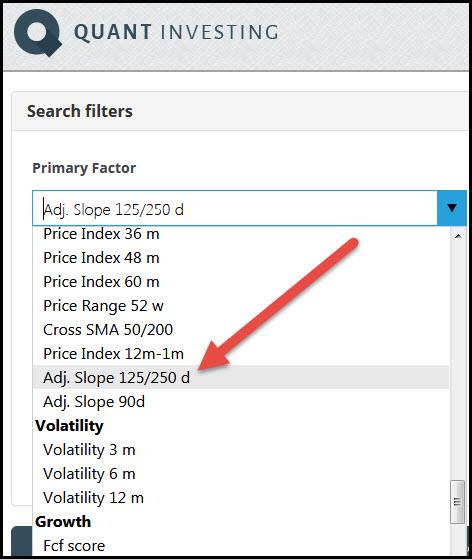How to select Adjusted Slope 125/250d as a primary screening factor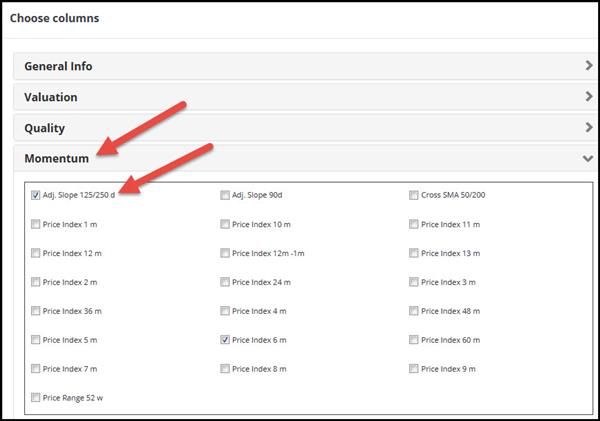How to select Adjusted Slope 125/250d as an output column

### Higher Adjusted Slope value is better

The higher the Adjusted Slope 125/250d value the better. It means the share price has increased a lot over an average of the past 125 and 205 trading days and that the calculated increase closely fits the real increase in the stock price.

You can select the best Adjusted Slope 125/250d values by setting the slider from 0% to 10% or by sorting the output column from high to low (to sort the column click on the column heading).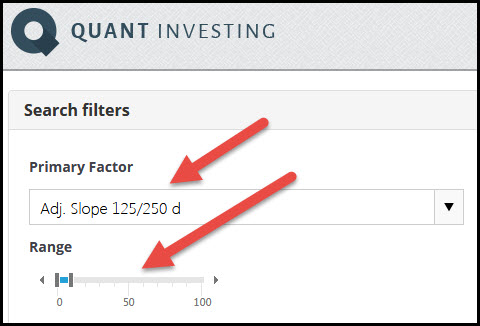How to select best Adjusted Slope 125/250d values

## Summary and conclusion

In summary the adjusted slope is a better momentum indicator you can use to find companies with a stock price that is moving up smoothly. It does this by removing companies with a stock price that has moved up in big jumps.

I am sure it is an indicator that will help you find even more profitable investment ideas as it is available on all the, more than 22,000 companies, in the screener.

PS To get unlimited access to the adjusted slope as well as over 110 other ratios and indicators you can use to find investment ideas sign up for the screener, it costs less than an inexpensive lunch for two.